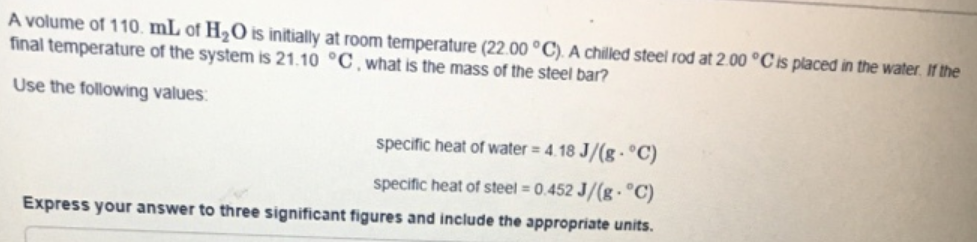# Problem: A volume of 110 mL of H2O is initially at room temperature (22.00°C) A chilled steel rod at 2.00°C is placed n the water. If the final temperature of the system is 21.10°C, what is the mass of the steel bar?Use the following values specific heat of water = 4.18 J/(g•°C) specific heal of steel = 0.452 J/(g•°C) Express your answer to three significant figures and include the appropriate units.

###### FREE Expert Solution
82% (388 ratings)###### Problem Details

A volume of 110 mL of H2O is initially at room temperature (22.00°C) A chilled steel rod at 2.00°C is placed n the water. If the final temperature of the system is 21.10°C, what is the mass of the steel bar?
Use the following values specific heat of water = 4.18 J/(g•°C) specific heal of steel = 0.452 J/(g•°C) Express your answer to three significant figures and include the appropriate units.Frequently Asked Questions

What scientific concept do you need to know in order to solve this problem?

Our tutors have indicated that to solve this problem you will need to apply the Thermal Equilibrium concept. You can view video lessons to learn Thermal Equilibrium. Or if you need more Thermal Equilibrium practice, you can also practice Thermal Equilibrium practice problems.